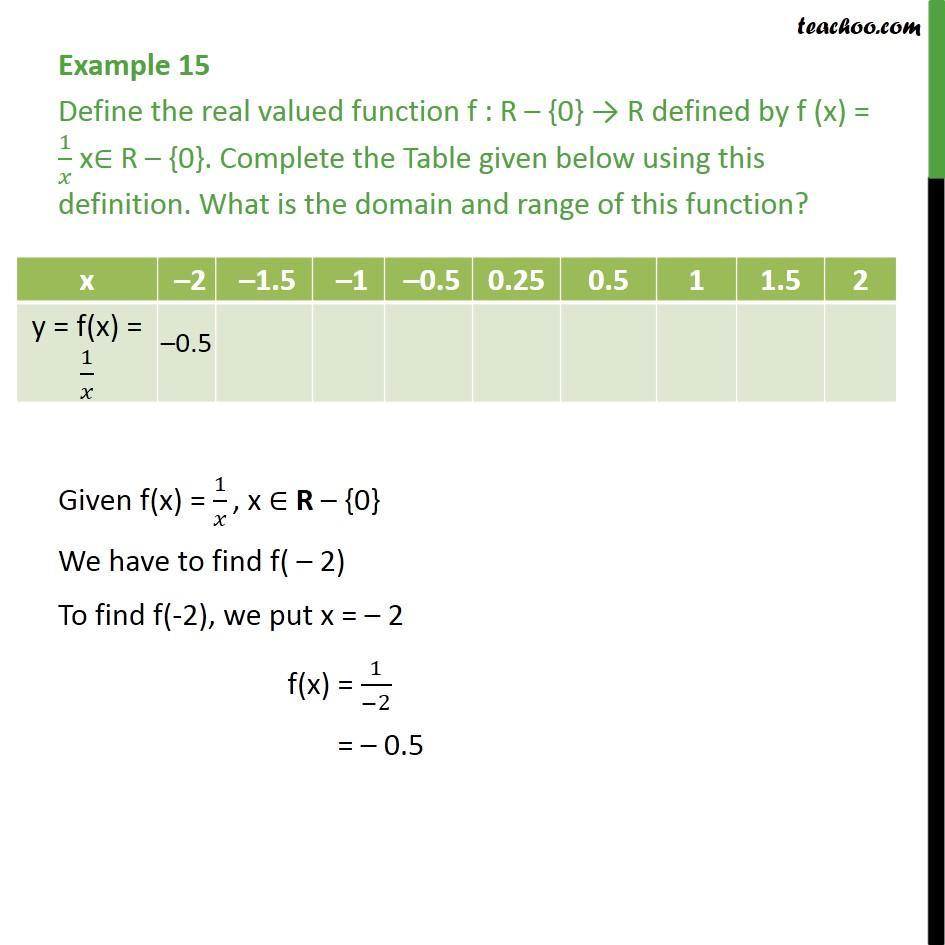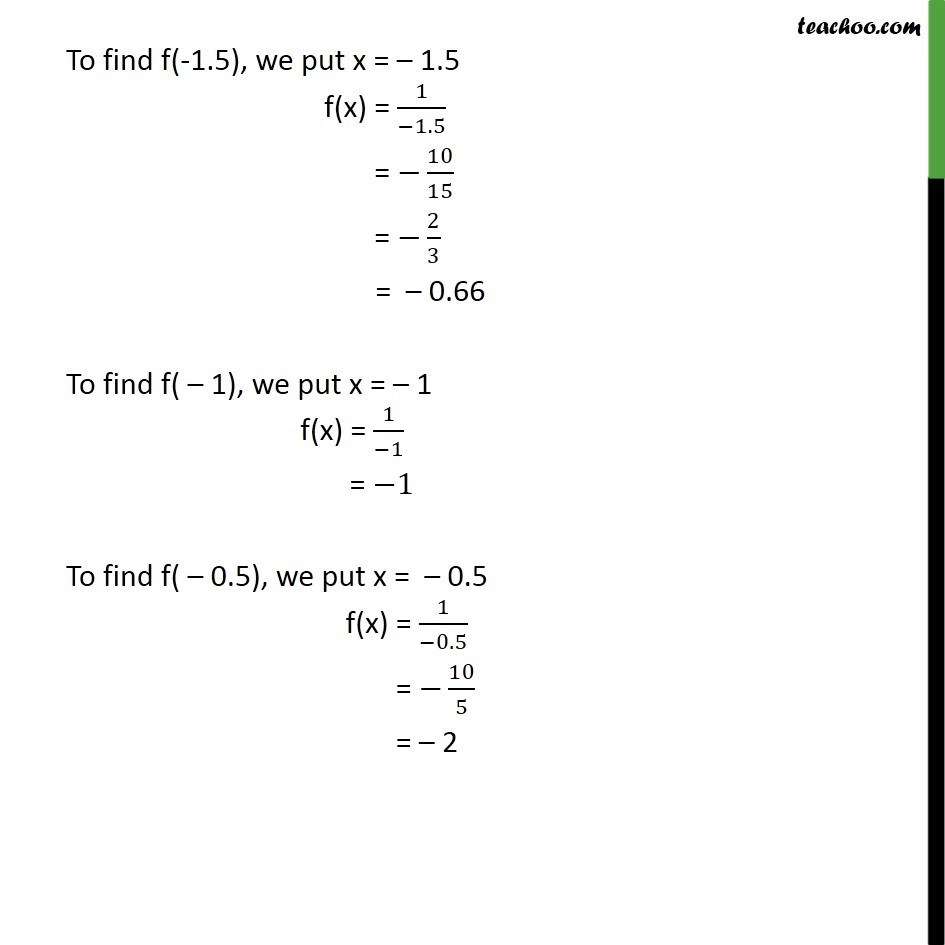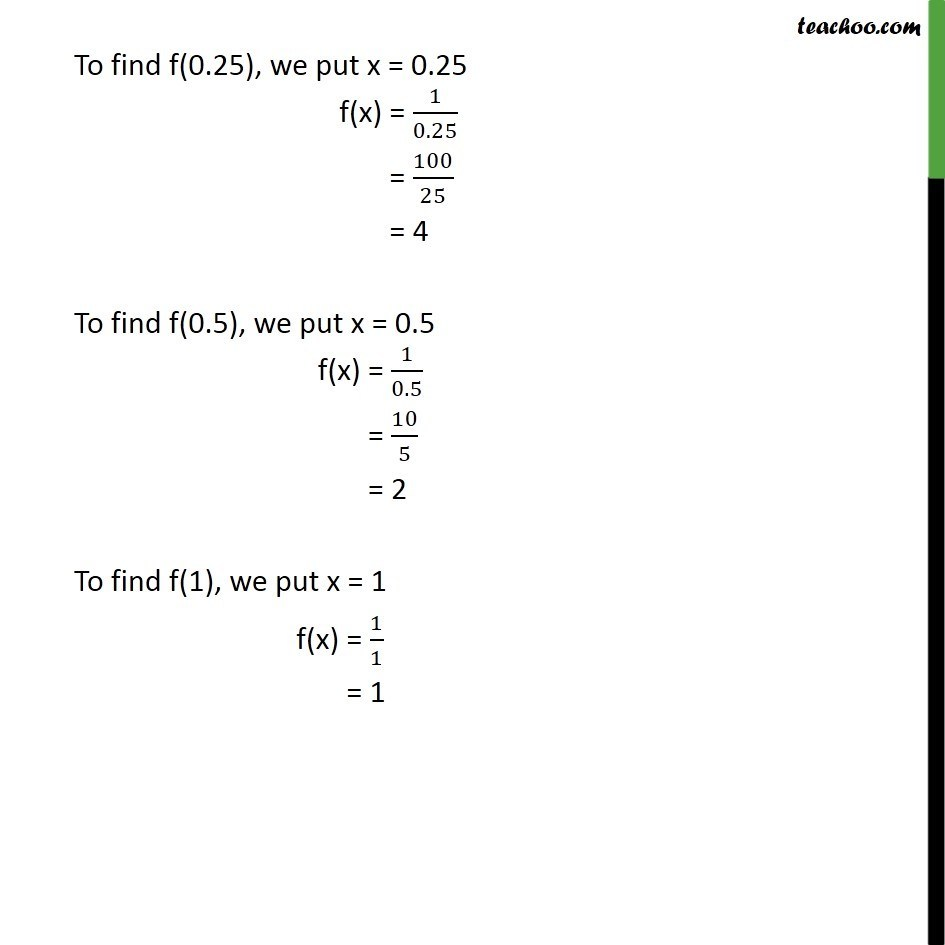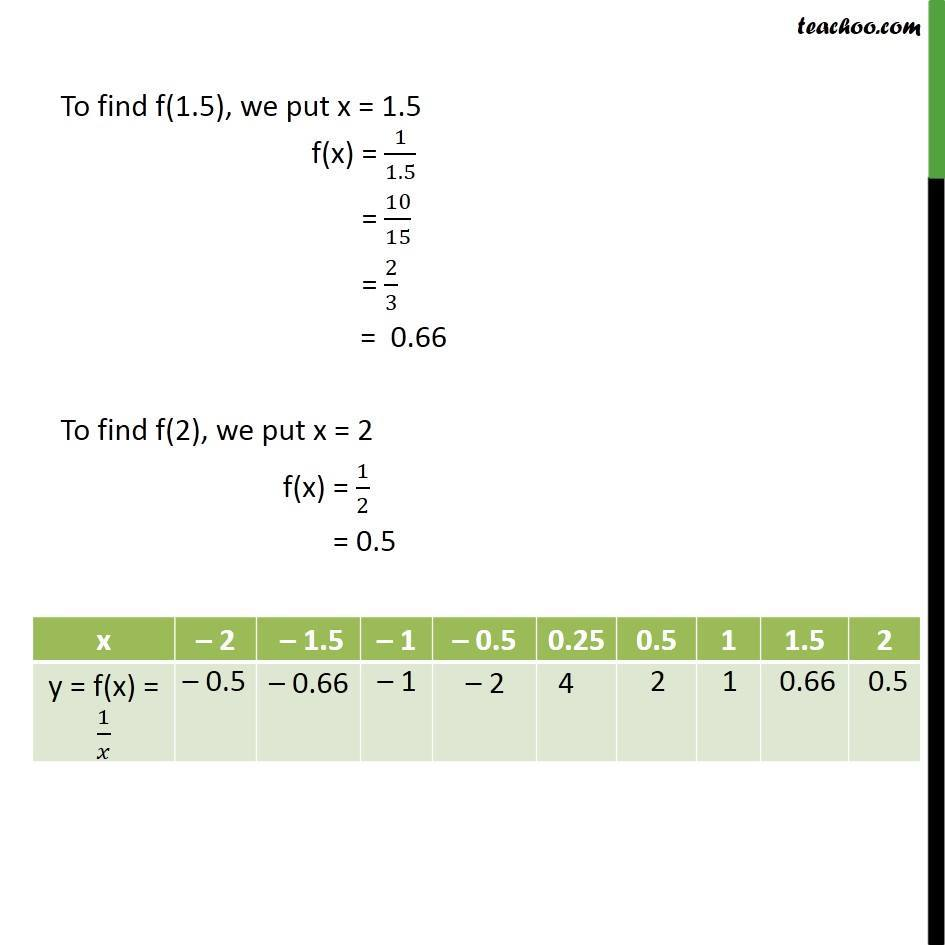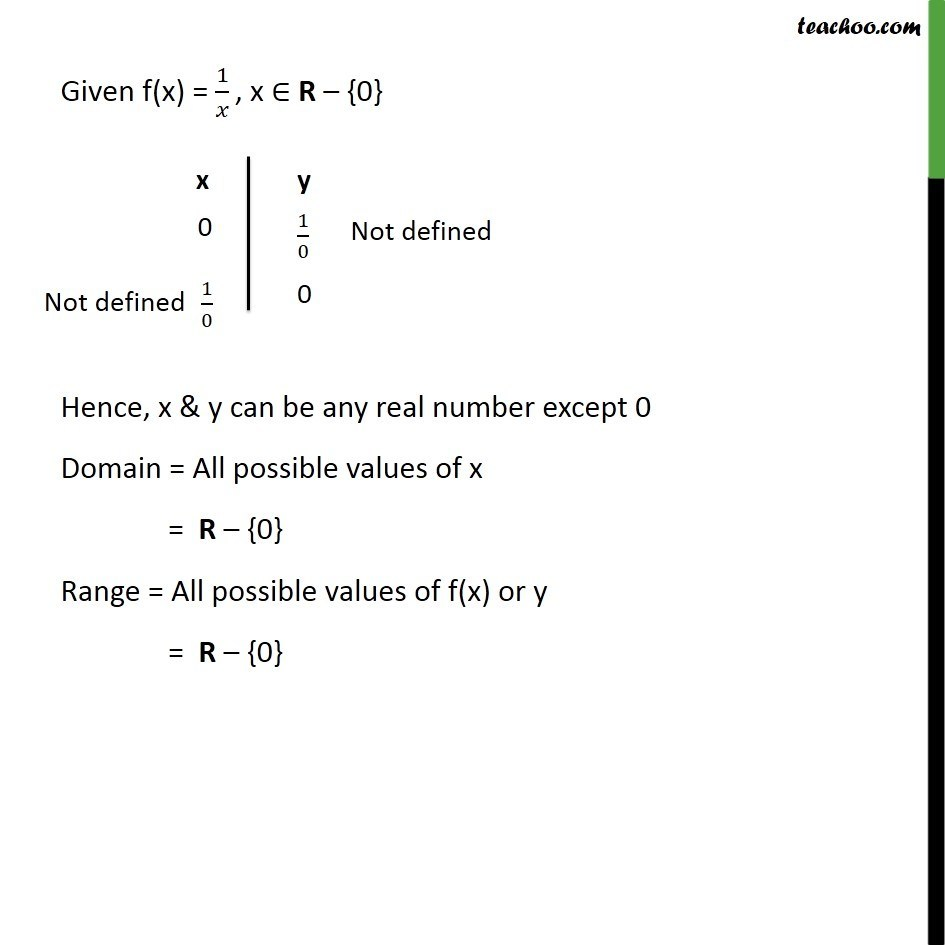1. Chapter 2 Class 11 Relations and Functions
2. Concept wise
3. Finding Domain and Range - By drawing graphs

Transcript

Example 15 Define the real valued function f : R – {0} → R defined by f (x) = 1/𝑥 x∈ R – {0}. Complete the Table given below using this definition. What is the domain and range of this function? Given f(x) = 1/𝑥 , x ∈ R – {0} We have to find f( – 2) To find f(-2), we put x = – 2 f(x) = 1/(−2) = – 0.5 To find f(-1.5), we put x = – 1.5 f(x) = 1/(−1.5) = −10/15 = −2/3 = – 0.66 To find f( – 1), we put x = – 1 f(x) = 1/(−1) = −1 To find f( – 0.5), we put x = – 0.5 f(x) = 1/(−0.5) = −10/5 = – 2 To find f(0.25), we put x = 0.25 f(x) = 1/0.25 = 100/25 = 4 To find f(0.5), we put x = 0.5 f(x) = 1/0.5 = 10/5 = 2 To find f(1), we put x = 1 f(x) = 1/1 = 1 To find f(1.5), we put x = 1.5 f(x) = 1/1.5 = 10/15 = 2/3 = 0.66 To find f(2), we put x = 2 f(x) = 1/2 = 0.5 Given f(x) = 1/𝑥 , x ∈ R – {0} Hence, x & y can be any real number except 0 Domain = All possible values of x = R – {0} Range = All possible values of f(x) or y = R – {0}

Finding Domain and Range - By drawing graphs